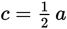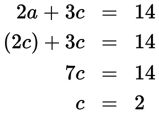# SAT Math Multiple Choice Question 800: Answer and Explanation

### Test Information

Question: 800

5. The total fare for two adults and three children on an excursion boat is \$14. If eachchild's fare is one-half of each adult's fare, what is the total cost for one adultand one child?

• A. \$4.00
• B. \$5.25
• C. \$6.00
• D. \$6.50

Explanation:

C

Difficulty: Medium

Category: Heart of Algebra / Systems of Linear Equations

Strategic Advice: Use the Kaplan Strategy for Translating English into Math to write two equations that represent the scenario. Use variables that make sense to help you stay organized (such as a for adult and c for child).

Getting to the Answer: Use the information given to write two equations in words first:

Two adult fares plus 3 child fares equals 14 → 2a + 3c = 14

One child fare equals one-half an adult fare →If you don't like working with fractions, multiply both sides of the second equation by 2 to get 2c = a. Then, plug 2c in for a into the first equation and solve for c:The cost of a child's fare is \$2, which is half the cost of an adult's fare. This means an adult's fare costs \$4. Don't fall for the trap answer, which is A. Add the two fares together to find that the total cost for one adult and one child is \$6, which is (C).